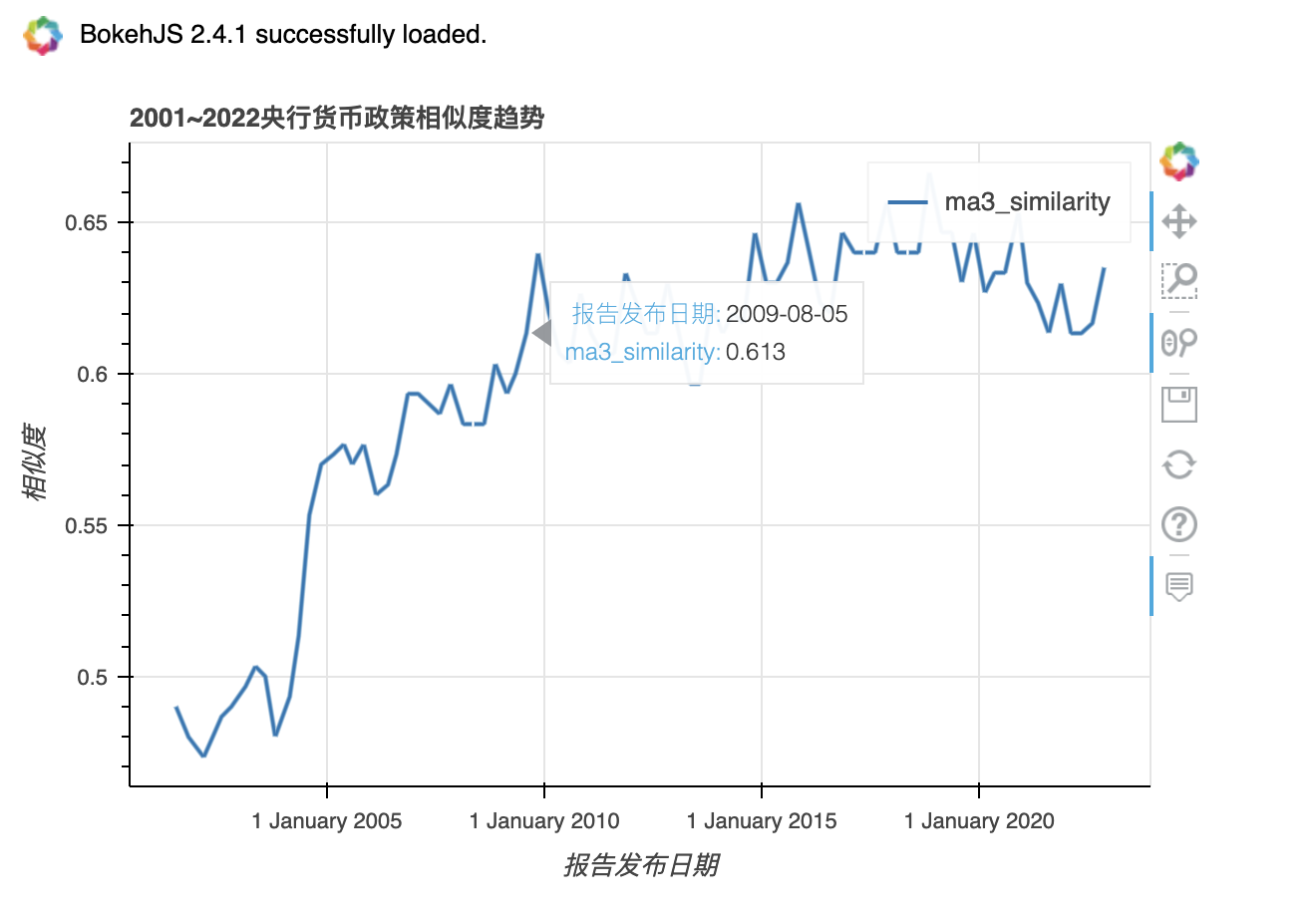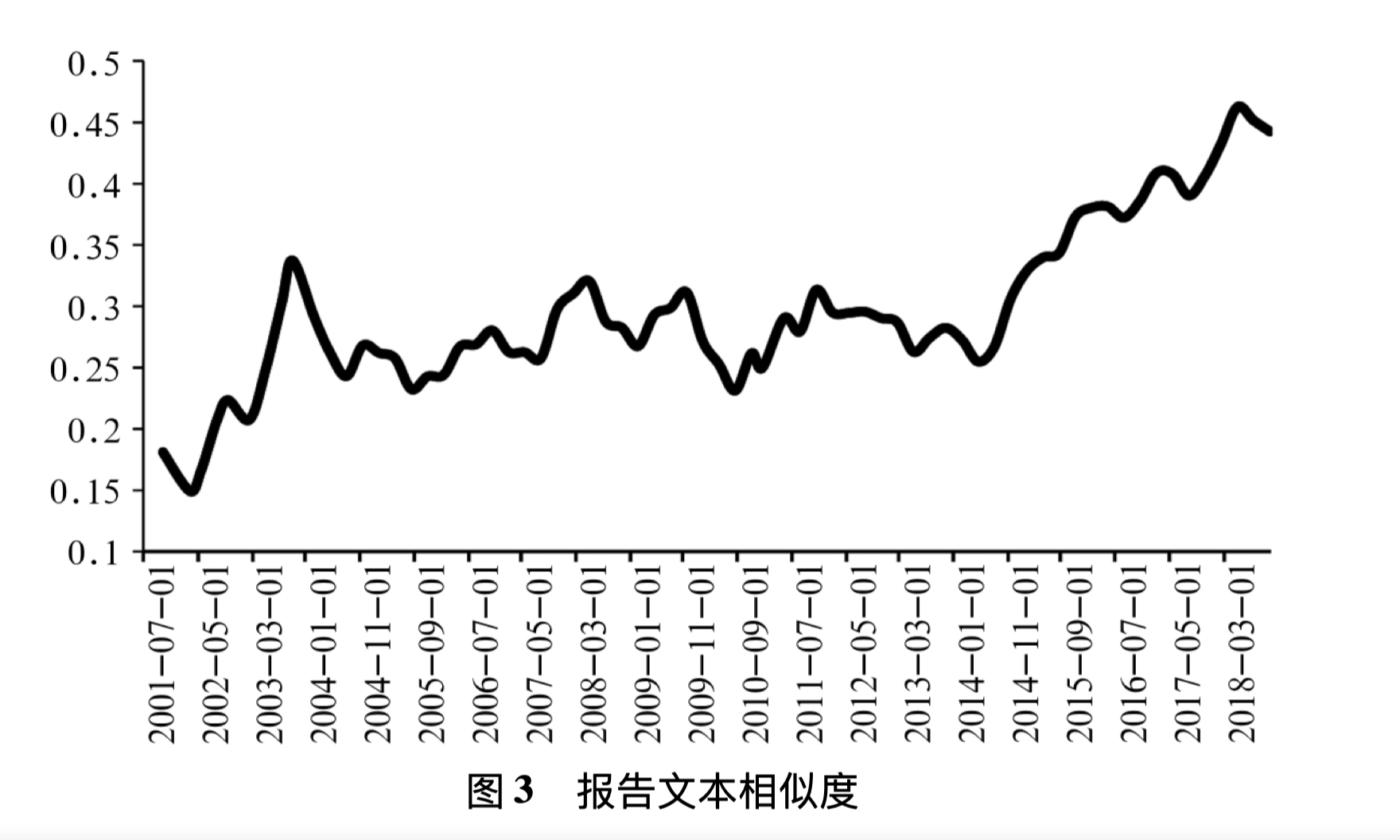1. 准备数据
2. 相似度计算
3. 可视化

## 1. 准备数据#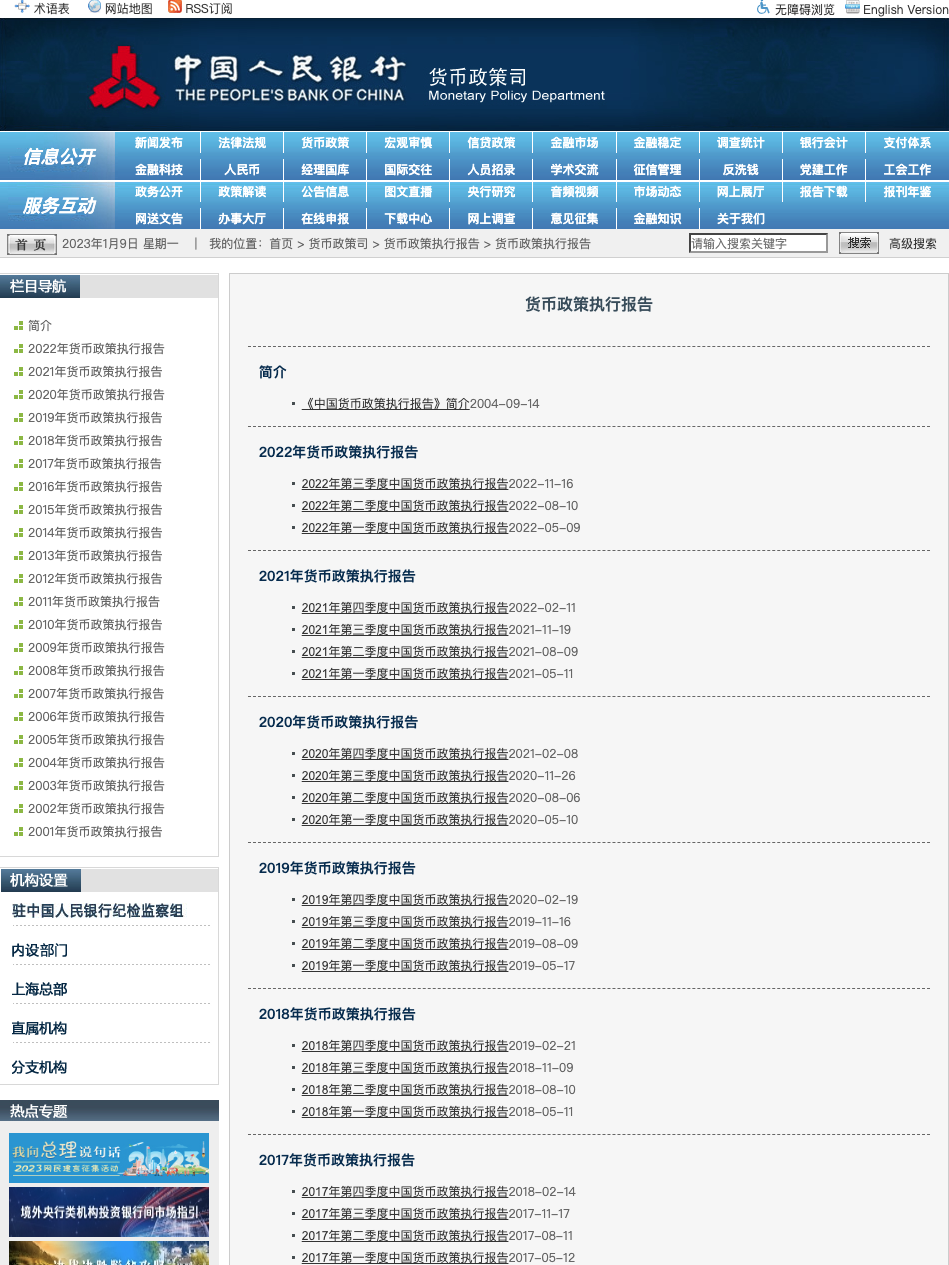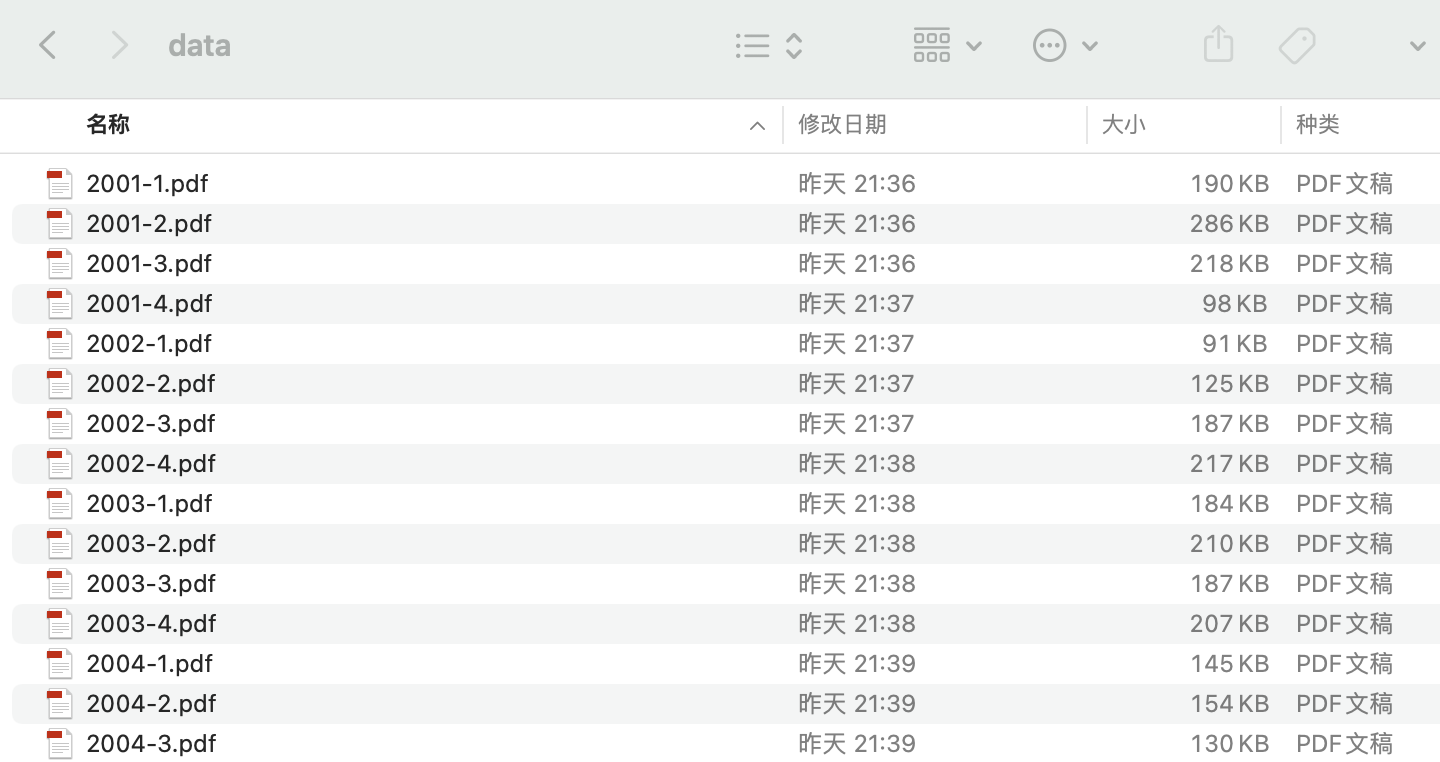import os
import csv

with open('pbc_reports.csv', 'a+', encoding='utf-8', newline='') as csvf:
#年份、季度、报告文本
fieldnames = ['year', 'q', 'text']
writer = csv.DictWriter(csvf, fieldnames=fieldnames)

pdfs = ['data/{}'.format(f) for f in os.listdir('data')]
for pf in pdfs:
data = {
'year':  pf.split('/')[-1][:4],
'q':  pf.split('/')[-1],
}
writer.writerow(data)


## 2. 读取数据#

import pandas as pd
import warnings
warnings.filterwarnings('ignore')



Run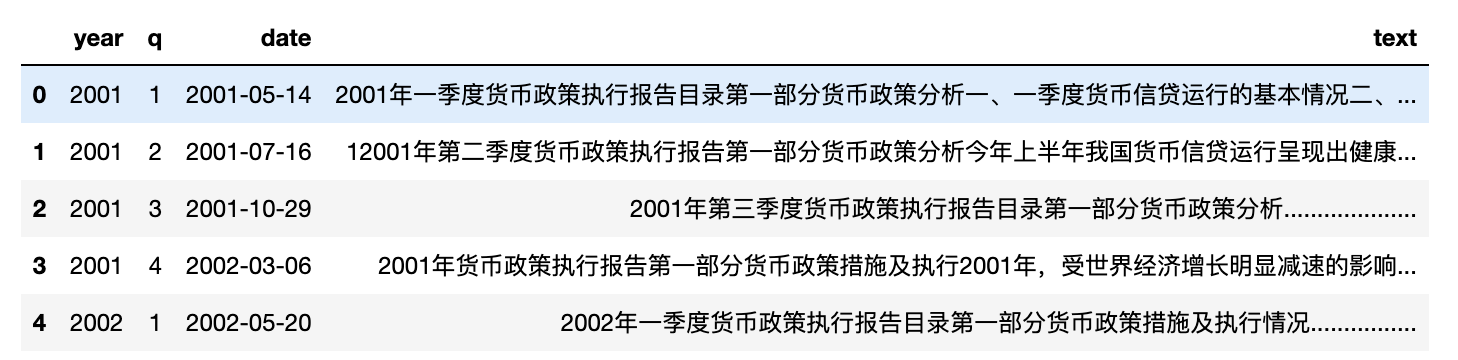#让每一行含有前后两个季度的报告文本
df['text2'] = df['text'].shift(1)
df = df[1:]


Run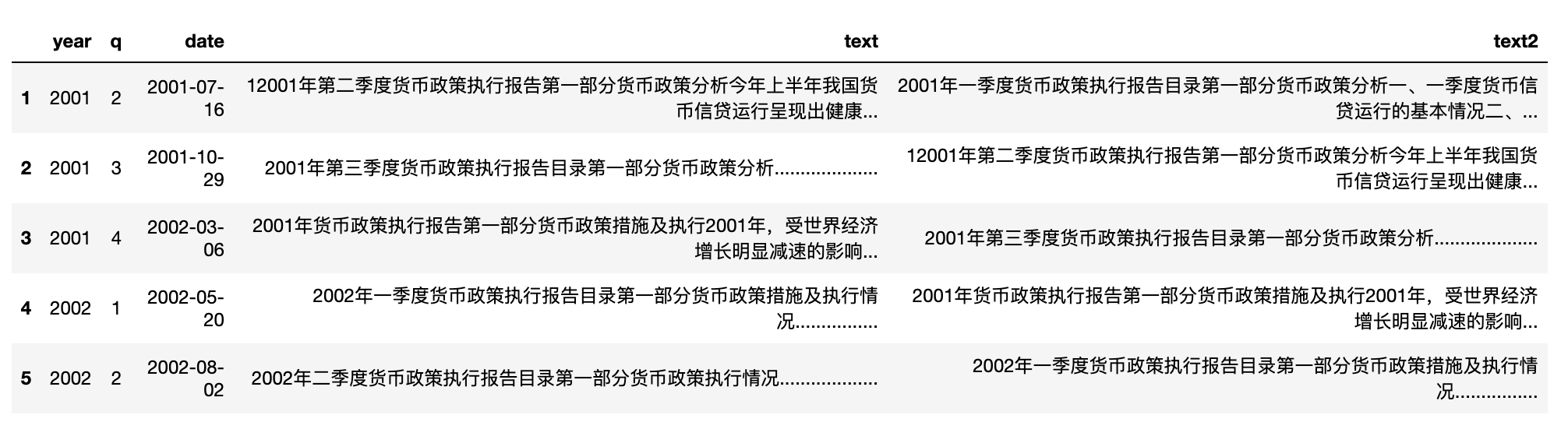## 3. 计算相似度#

import cntext as ct

def cosine_similarity(row):
#row 为 pd.Series 类型数据，类似于字段
try:
sim = ct.cosine_sim(row['text'], row['text2'])
return float(sim)
except:
#异常标记为1
return 1

#计算结果存储到 similarity 字段中
df['similarity'] = df.apply(lambda row: cosine_similarity(row), axis=1)


Run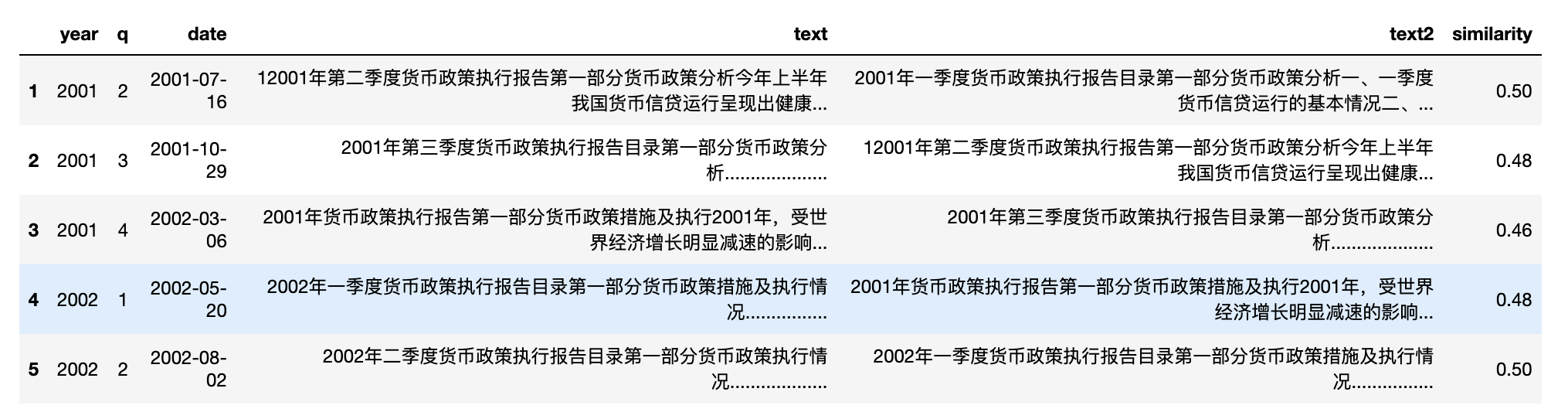## 4. 绘制折线图#

• kind 图表类型，折线图line
• x 横轴字段
• y 纵轴字段
• xlabel 横轴标签
• ylabel 纵轴标签
• title 图标题
import pandas_bokeh

pandas_bokeh.output_notebook()

#选择折线图line
#
df.plot_bokeh(kind = 'line',
x = 'date',
y = 'similarity',
title = '2001~2022央行货币政策相似度趋势',
xlabel = '报告发布日期',
ylabel = '相似度')


Run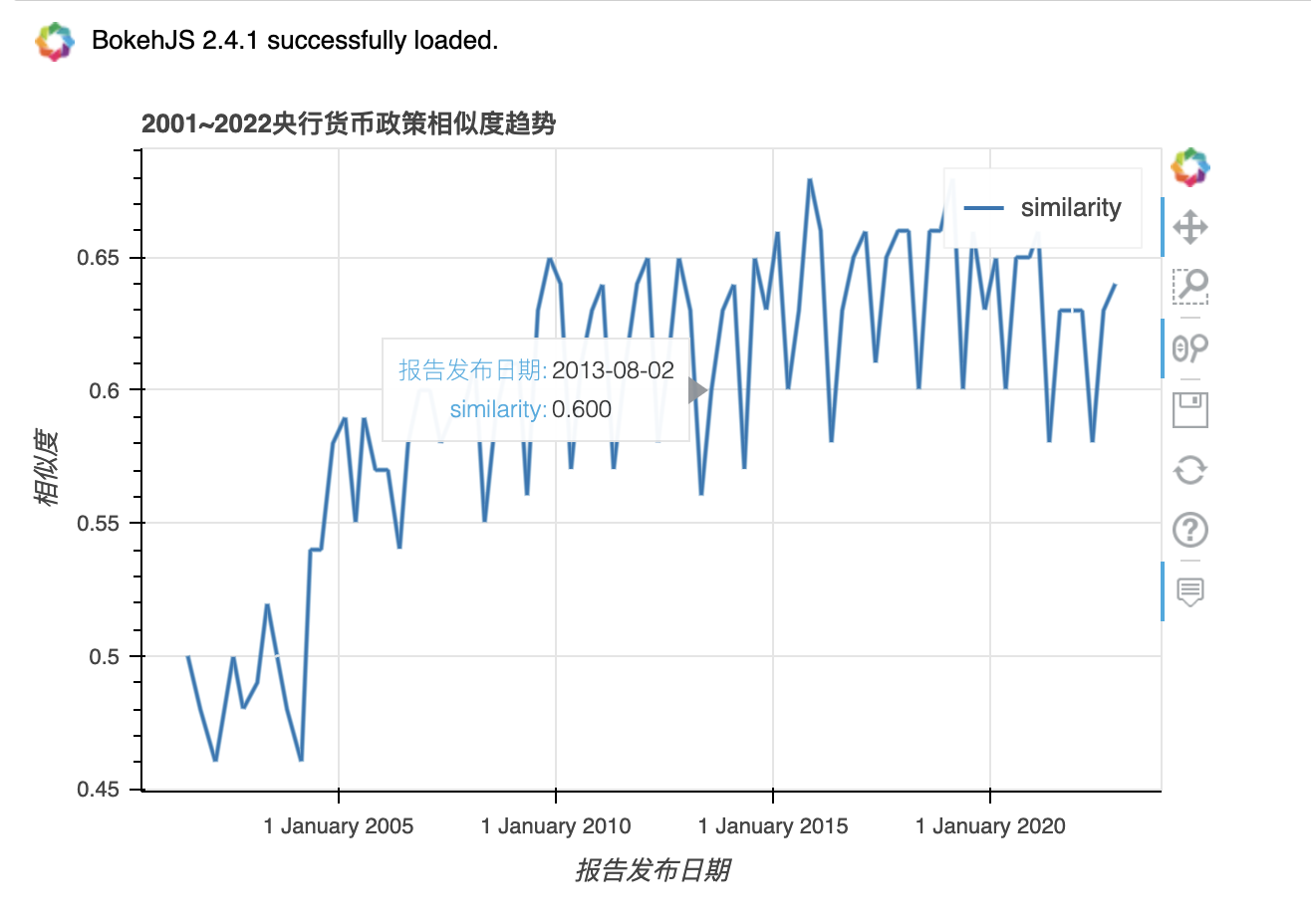import pandas_bokeh

pandas_bokeh.output_notebook()

#三季度移动平均线
df['ma3_similarity'] = df['similarity'].rolling(window=3, center=True, min_periods=1).mean()

df.plot_bokeh(kind = 'line',
x = 'date',
y = 'ma3_similarity',
title = '2001~2022央行货币政策相似度趋势',
xlabel = '报告发布日期',
ylabel = '相似度')


Run